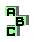GOB POINT

 r49 orthic axis, circumcircle r51 Clawson point, point X(33), point X(55) r129 orthic axis, circumcircle, circumcenter, inverse points d point X(468) r480 isogonal points, symmedian point, orthic triangle r728 Exeter point, complement, point X(468), ninecircle, orthocentroidal circle, inverse points r907 intouch triangle, orthic triangle, perspective r936 pedal triangle, circumcircle r1092 Parry point, point X(110), point X(858), concyclic points r1101 orthoassociate points, point X(858) r1239 orthocenter, pedal triangle, orthic triangle, homothetic triangles r1582 orthic triangle r1631 altitude feet, circumcircle, tangent circles r1845 circumcircle, tangent circles r1886 circumcevian triangle, tangential triangle, perspective, centroid r1892 retrocenter, isogonal points r1977 altitude feet, isogonal points, centroid r2024 altitude feet, altitudes, ninecircle